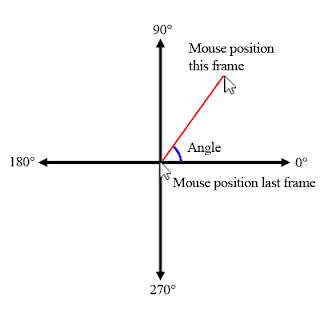### I Never Knew Moving a Lever Could Be So Hard

In the Zork games, there are switches/twist knobs/turn-tables:

These are all controlled by the Lever control:

control:624 lever {
descfile(knocker.lev)
cursor(handpt)
}


The knocker.lev file looks like this:

animation_id:631~
filename:te2ea21c.rlf~
skipcolor:0~
anim_coords:200 88 343 315~
mirrored:1~
frames:11~
elsewhere:0 0 511 319~
out_of_control:0 0 511 319~
start_pos:0~
hotspot_deltas:42 39~
0:241 252 D=1,90 ^=P(0 to 1) P(1 to 0) P(0 to 1) P(1 to 0) E(0)~
1:234 260 D=2,90 D=0,270 ^=P(1 to 0) E(0)~
2:225 258 D=3,90 D=1,270 ^=P(2 to 0) P(0 to 1) P(1 to 0) E(0)~
3:216 255 D=4,90 D=2,270 ^=P(3 to 0) P(0 to 1) P(1 to 0) E(0)~
4:212 234 D=5,90 D=3,270 ^=P(4 to 0) P(0 to 2) P(2 to 0) E(0)~
5:206 213 D=6,90 D=4,270 ^=P(5 to 0) P(0 to 3) P(3 to 0) E(0)~
6:212 180 D=7,90 D=5,270 ^=P(6 to 0) P(0 to 3) P(3 to 0) E(0)~
7:214 147 D=8,90 D=6,270 ^=P(7 to 0) P(0 to 4) P(4 to 0) E(0)~
8:222 114 D=9,90 D=7,270 ^=P(8 to 0) P(0 to 5) P(4 to 0) E(0)~
9:234 106 D=10,90 D=8,270 ^=P(9 to 0) P(0 to 5) P(4 to 0) E(0)~
10:234 98 D=9,270~

• animation_id is unused.
• filename refers to the animation file used.
• skip color is unused.
• anim_coords refers to the location the control will be rendered
• mirrored says that the reverse of the animation is appended to the end of the file. Ex: 0, 1, 2, 3, 3, 2, 1, 0
• frames refers to how many animation frames there are (If mirrored = 1, frames = animationFile::frameCount / 2)
• elsewhere is unused
• out_of_control is unused
• start_pos refers to the first animation frame used by the control
• hotspot_deltas refers to the width and height of the hotspots used to grab a control with the mouse

The last section is a bit tricky. It’s formatted like so:

[frameNumber]:[hotspotX] [hotspotY] D=[directionToFrame],[directionAngle] .....(potentially more directions) ^=P([from] to [to]) P([from] to [to]) ... (potentially more return paths) E(0)~

• frameNumber corresponds the animationFile frame that should be displayed when the lever is in that state
• hotspotX is the X coordinate of the hotspot rectangle in which the user can grab the control
• hotspotY is the Y coordinate of the hotspot rectangle in which the user can grab the control

D refers to “Direction”. Let’s say we’re at frame 0. D=1,90 means: “To get to frame 1, the mouse needs to be moving at a 90 degree angle.” (I’ll cover how the angles work in a bit)

P refers to “Path”. This is what frames should be rendered after the user lets go of a control. For example, lets say we let go of the knocker at frame 6. The .lev file reads: ^=P(6 to 0) P(0 to 3) P(3 to 0). This says to render every frame from 6 to 0, then every frame from 0 to 3, then every frame from 3 to 0. So written out:

6, 5, 4, 3, 2, 1, 0, 0, 1, 2, 3, 3, 2, 1, 0

This allows for some cool effects such as the knocker returning to the lowest position and bouncing as though it had gravity.

So what is that angle I was talking about? It refers to the direction the mouse is moving while the user is holding down left mouse button.So let’s go over a typical user interaction:

1. User hovers over the control. The cursor changes to a hand.
2. User presses down the left mouse button
3. Test if the mouse is within the current frame’s hotspot
4. If so, begin a drag:
1. Calculate the distance between the last mouse position and the current
2. If over 64 (a heuristic), calculate the angle. (Only calculating the angle when we’re sufficiently far from the last mouse position saves calculations as well as makes the lever less “twitchy”
3. Test the angle against the directions
4. If one passes, render the new frame
5. User moves a couple more times
6. User releases the left mouse button
1. Follow any return paths set out in the .lev file

And that’s it! Let me know if you have any questions or comments. The full source code can be found here and here.

Until next time, happy coding! :)